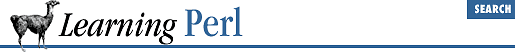home | O'Reilly's CD bookshelfs | FreeBSD | Linux | Cisco | Cisco Exam

### A.16 Chapter 17, User Database Manipulation

1. Here's one way to do it:

```

dbmopen(%ALIAS, "/etc/aliases", undef) ||
die "No aliases!: \$!";
while ((\$key,\$value) =

each(%ALIAS)) {
chop(\$key,\$value);
print "\$key \$value\n";
}```

The first line opens the aliases DBM. (Your system may keep the aliases DBM in /usr/lib/aliases instead - try that if this doesn't work.) The ``` while``` loop steps through the DBM array. The first line within the loop chops off the NUL character from the end of the key and the value. The final line of the loop prints out the result.

2. Here's one way to do it:

```# program 1:
dbmopen(%WORDS,"words",0644);
while (

<>) {
foreach \$word (split(/\W+/)) {
\$WORDS{\$word}++;
}
}
dbmclose(%WORDS);```

The first program (the writer) opens a DBM in the current directory called ``` words``` , creating files named words.dir and words.pag . The ``` while``` loop grabs each line using the diamond operator. This line is split apart using the ``` split``` operator, with a delimiter of ``` /\W+/``` , meaning nonword characters. Each word is then counted into the DBM array, using the ``` foreach``` statement to step through the words:

```# program 2:
dbmopen(%WORDS,"words",undef);
foreach \$word (sort { \$WORDS{\$b}

<=> \$WORDS{\$a} } keys %WORDS) {
print "\$word \$WORDS{\$word}\n";
}
dbmclose(%WORDS);```

The second program opens a DBM in the current directory called ``` words``` . That complicated looking ``` foreach``` line does most of the dirty work. The value of ``` \$word``` each time through the loop will be the next element of a list. The list is the sorted keys from ``` %WORDS``` , sorted by their values (the count) in descending order. For each word in the list, we print the word and the number of times the word has occurred.A.15 Chapter 16, System Database AccessA.17 Chapter 18, Converting Other Languages to Perl